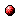# Model predictive control for randomly switching max-plus-linear systems using a scenario-based algorithm

Reference:
T. van den Boom and B. De Schutter, "Model predictive control for randomly switching max-plus-linear systems using a scenario-based algorithm," Proceedings of the 49th IEEE Conference on Decision and Control, Atlanta, Georgia, pp. 2298-2303, Dec. 2010.

Abstract:
Switching max-plus-linear (SMPL) systems are discrete event systems that can switch between different modes of operation. In each mode the system is described by a max-plus-linear state equation and a max-plus-linear output equation, with different system matrices for each mode. The switching between from one mode to the other is a stochastic process. In the model predictive control (MPC) formulation stability is enforced by additional constraints. To reduce the computational complexity we use an algorithm based on scenario generation for such stochastic SMPL systems.Corresponding technical report: pdf file (162 KB)
Note: More information on the pdf file format mentioned above can be found here.

Bibtex entry:

@inproceedings{vanDeS:10-050,
author={T. van den Boom and B. {D}e Schutter},
title={Model predictive control for randomly switching max-plus-linear systems using a scenario-based algorithm},
booktitle={Proceedings of the 49th IEEE Conference on Decision and Control},